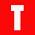Sunday, May 17, 2009

# non-static method ... cannot be referenced from a static context

on
If you try to access a non static member of a class from a static context, you will see ‘non-static method … cannot be referenced from a static context’. For example, you might have tried to call a non static method from a static method. Bear in mind, you can access a static member (variables, methods etc) from both static and non-static context but cannot access non-static member from a static context.

See the following example code:

public class NonStaticMethodCannotBeReferencedFromAStaticContext
{
private double firstNumber;
private double secondNumber;
private double result;

public void setFirstNumber(double firstNubmer)
{
this.firstNumber=firstNumber;
}

public void setSecondNubmer(double secondNumber)
{
this.secondNumber=secondNumber;
}

public double add()
{
return firstNumber+secondNumber;
}

public double substract()
{
return firstNumber-secondNumber;
}

public static void main(String args[])
{
setFirstNumber(10.35);// this is a non-static method but referenced from static main method
setSecondNubmer(20.97);
System.out.println("The addition is "+add());
}

}

For the solution you can:
i) declare the non-static member as static and access them from the static context (not always; be careful, this may create other problems)
ii) Create an instance of the class where the non-static members are and then reference the non-static members by the created instance or object.

Browse http://javatech-stuff.blogspot.com/2007/11/differences-between-java-terms.html for understanding the differences between static and non-static member.

Now see the solution below:

public class NonStaticMethodCannotBeReferencedFromAStaticContextSolved
{
private double firstNumber;
private double secondNumber;
private double result;

public void setFirstNumber(double firstNubmer)
{
this.firstNumber=firstNumber;
}

public void setSecondNubmer(double secondNumber)
{
this.secondNumber=secondNumber;
}

public double add()
{
return firstNumber+secondNumber;
}

public double substract()
{
return firstNumber-secondNumber;
}

public static void main(String args[])
{
NonStaticMethodCannotBeReferencedFromAStaticContextSolved object=new NonStaticMethodCannotBeReferencedFromAStaticContextSolved();
object.setFirstNumber(10.35);// accessed by the instance named object
object.setSecondNubmer(20.97);
System.out.println("The addition is "+object.add());
}

}

If the above solutions do not work for you. Please comment below giving your code. I'll try to respond.
Share:

#### 1 comments:

1.public static void main(String args[])
{
setFirstNumber(10.35);// this is a non-static method but referenced from static main method
setSecondNubmer(20.97);
System.out.println("The addition is "+add());
}

}

http://tehapps.com/